Next: Magnetostatics in Magnetic Media Up: Magnetostatic Fields Previous: Localized Current Distribution

# Exercises

1. Consider two thin current loops. Let loops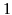and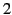carry the currents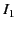and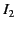, respectively. The magnetic force exerted on loop 2 by loop 1 is [see Equation (616)]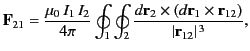where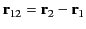. Here,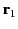and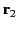are the position vectors of elements of loopsand, respectively. Demonstrate that the previous expression can also be written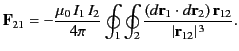Hence, deduce that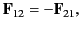in accordance with Newton's third law of motion.

2. Consider the two current loops discussed in the previous question. The magnetic field generated at a general position vector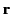by the current flowing around loopis [see Equation (614)]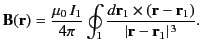Demonstrate that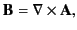where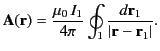Show that the magnetic flux passing through loop, as a consequence of the current flowing around loop, is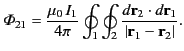Hence, deduce that the mutual inductance of the two current loops takes the form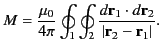3. The vector potential of a magnetic dipole of moment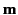is given by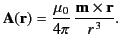Show that the corresponding magnetic field is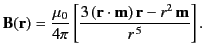4. Demonstrate that the torque acting on a magnetic dipole of momentplaced in a uniform external magnetic field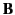is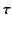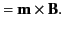Hence, deduce that the potential energy of the magnetic dipole is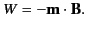5. Consider two magnetic dipoles,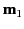and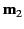. Suppose thatis fixed, whereascan rotate freely in any direction. Demonstrate that the equilibrium configuration of the second dipole is such that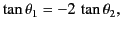where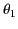and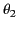are the angles subtended byand, respectively, with the radius vector joining them.Next: Magnetostatics in Magnetic Media Up: Magnetostatic Fields Previous: Localized Current Distribution
Richard Fitzpatrick 2014-06-27